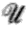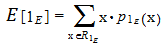# Indicator Function: Definition, Examples

## Disambiguation

“Indicator function” can mean different things depending on where you read about it:

1. In probability and set theory: A random variable for an event that equals 1 when the event happens and 0 when the event does not happen.
2. In statistics: A synonym for a characteristic function, which completely defines a probability distribution.

According to Professor Greg Lawler of the University of Chicago1, the term “indicator function” is the first definition: a random variable that takes on a value of 0 or 1.

“The corresponding function in analysis is often called the characteristic function and denoted χE. Probabilists never use the term characteristic function for the indicator function because the term characteristic function has another meaning. The term indicator function has no ambiguity.” ~ Greg Lawler.

## What is an Indicator Function in Probability?

Indicator functions are useful “switches”, simplifying probability statements to “Yes” or “No” statements. The concept similar to how an if() statement works in computer programming.

The indicator function, Ia (or IE) is defined on the whole space(which is the universal set in set theory). This simple function has just two values: 0 and 1.

• 0 = the event happens,
• 1 = the event does not happen.

For example, let’s say you were interested in knowing how many people voted for Alvin Brown for mayor of Jacksonville.

• A “1” means the event happened (they voted for him),
• A “0” means the event didn’t happen (they did not vote for him).

## Indicator Functions and Expectation

The expectation of the indicator function for an event is the probability of that event.
Why? The expected value for a random variable is the sum of the events multiplied by their probabilities. For example, if you wanted to find the expected value for rolling a single die, the expected value is:

E(X) = x * P(x) = 1(1/6) + 2(1/6) + 3(1/6) + 4(1/6) + 5(1/6) + 6(1/6) = 3.5.

In the same way, the expectation for an indicator function is also the sum of the probabilities. In notation, that’s:The two probabilities involved with indicator functions are:

• the event happens (1),
• or the event doesn’t happen (0), giving:

References:
(1) Lawler, G. (2016). Probability Notes.

CITE THIS AS:
Stephanie Glen. "Indicator Function: Definition, Examples" From StatisticsHowTo.com: Elementary Statistics for the rest of us! https://www.statisticshowto.com/indicator-function/